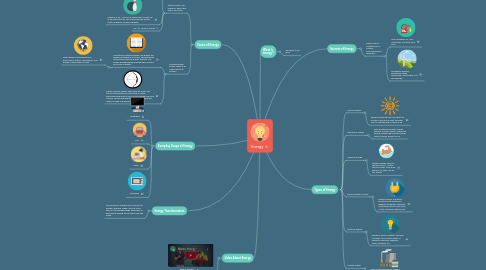# EnergyAliza MALIK
Get Started. It's FreeEnergy## 1. Forms of Energy

### 1.1. Kinetic Energy: The energy an object has due to its motion.

1.1.1. Factors that affect KE : If the mass and velocity of an object increases, the kinetic energy also increases.

1.1.2. Example of KE : If you roll a bowling ball & a golf ball at the same velocity, the bowling ball has greater kinetic energy as its mass is greater.

1.1.3. KE =1/2 x mass x velocity ^2

### 1.2. Potential Energy: Energy stored in an object due to its position

1.2.1. Gravitational Potential Energy: The greater the height and weight of an object, the greater the gravitational potential energy. Example: The Earth's gravitational PE is greater than a book's as its mass is greater

1.2.1.1. When energy is transferred to an Earth-object system , gravitational field energy of the system increase.

1.2.2. Elastic Potential Energy: The energy an object has due to being deformed. This energy is usually associated with objects that can be stretched like bow & arrow. Factors that affect this energy: material the object is made of & amount of deformation

## 6. Sources of Energy

### 6.1. Energy can be classified into 2 sources: Non-renewable & renewable

6.1.1. Non-renewable: Oil, coal, natural gas, and other fossil fuels

6.1.2. Renewable: Biomass, geothermal power, hydropower, solar energy, and wind energy.

## 7. Types of Energy

### 7.1. Thermal Energy

7.1.1. Energy transferred from one object to another in the form of heat. Example: The sun radiates heat to warm us up.

### 7.2. Mechanical Energy

7.2.1. Sum of potential & kinetic energy. Example: Football thrown. Higher the football, greater the potential energy. Faster it moves, greater its K.e.

### 7.3. Chemical Energy

7.3.1. Potential energy stored in chemical bonds. Usually stored in foods. After done eating, your body can use this energy.

### 7.4. Electromagnetic Energy

7.4.1. Travels in waves. The waves consist of both electrical & magnetic properties. Examples: microwaves used to cook food, X-rays, ultraviolet radiation, etc.

### 7.5. Electrical Energy

7.5.1. Energy of electric charges. This type of energy can be either kinetic or potential. Examples: Lightning, radios, batteries, etc.

### 7.6. Nuclear Energy

7.6.1. Type of potential energy stored in the nucleus of an atom. Released during a nuclear reaction. Example: Nuclear fission occurs when a nucleus splits. Nuclear power plants use fission to create electricity.